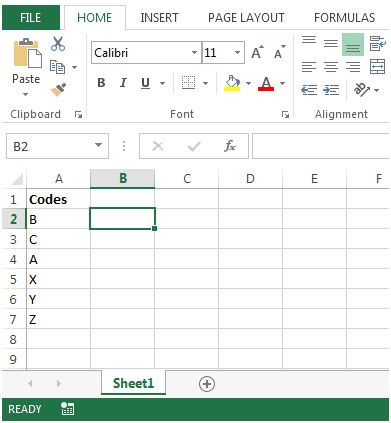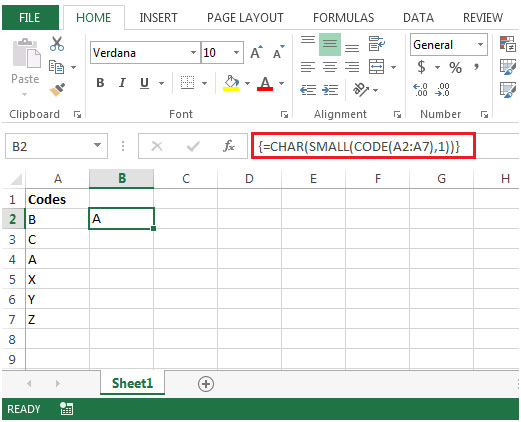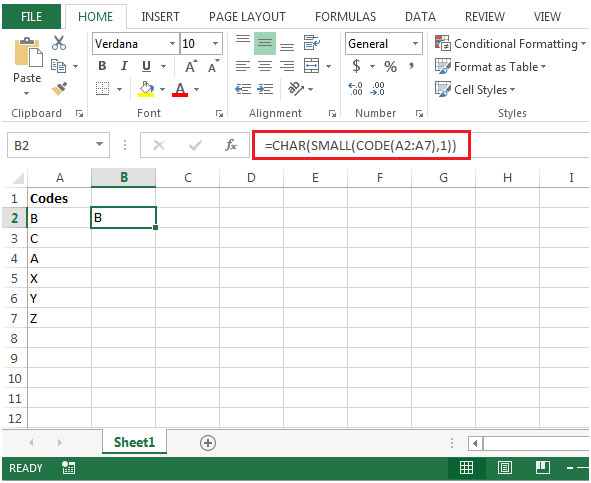# Find the Alphabetically Lowest Letter in a List in Microsoft Excel 2010

In this article, we will learn how to find the alphabetically lowest letter in a list in Microsoft Excel 2010.

If you want to find the alphabetically lowest letter in a list, you can use CHAR, CODE & SMALL functions together to get the output.

CHAR: Returns the character specified by the code number from the character set for your computer.

Syntax: =CHAR(number)

number: Itis a number between 1 and 255, specifying which character you want.

CODE: Returns a numeric code for the first character in a text string, in the character set used by your computer.

Syntax: =CODE(text)

text: The text for which you want the code of the first character.If there is more than one character, the function will return the ASCII value for the first character and ignore rest of the characters.

SMALL: Returns the k-th smallest value in a data set. For example: the fifth smallest number from a list of 20 items.

Syntax: =SMALL(array,k)

array: Itis an array or range of cells in a list of data for which you want to find the k-th smallest value.

k: Itis the kth position from smallest value to return in the array or range of cells.

Let us take an example:

• We have a list of alphabets which represent some Codes.• We will enter the formula in cell B2{=CHAR(SMALL(CODE(A2:A7),1))}with CTRL + SHIFT + ENTER• Evaluating the Code function (using F9 key), we will get {66;67;65;88;89;90}& out of this number list, 65 is the smallest value. The Code function then pass this range of values to Small function as a first argument, 1 is the kth smallest value.
• The value pass by Small function is 65 is then converted by Char function & returns A as our output.
• If we do not enclose this formula with curly brackets then it will show wrong result.
• If we use formula as =CHAR(SMALL(CODE(A2:A7),1))• The above formula has returned B, which is a wrong answer.

1.Abubakar Ansari

The code is starting from LT-101 to LT-102, LT-103..........in the colums or cell A so plz help me how can I type this method shortly.

2.how to convert numeric into text

•In context of this article, you can use CHAR function in excel. CHAR(number). and if you want to convert text format ,

select number containing cells and press CTRL+1. and select text.

3.I record daily stock prices on 12 worksheets (corresponding to the months in a year). I would like to create a summary page where the most recent prices would be found by referencing the individual worksheets. Any ideas?

Terms and Conditions of use

The applications/code on this site are distributed as is and without warranties or liability. In no event shall the owner of the copyrights, or the authors of the applications/code be liable for any loss of profit, any problems or any damage resulting from the use or evaluation of the applications/code.